## Monday, September 24, 2012

### On the growth laws of cell sheets

I usually start a new scientific project without studying the published literature of the domain in advance, because knowing that almost everything has already been done (which is invariably the case) spoils my explorative mood and blocks my creativity. As a result, when it comes to a publication of the results, I have a hard time to find a decent number of relevant citations. Very often, then, the referees criticize my short citation list and - as one of them put it approximately - the 'strange disconnection of my manuscript from the history'.

I was therefore pleased to read the following passage in Edward de Bono's creativity book 'Think! Before It's Too Late (2009)':
A very eminent scientist once asked me why I did not have lists of references in my books. I replied that it was because the ideas were mine and not obtained through scholarly research into other people's work. He told me that nevertheless I should 'fake' a reference list, whether or not I had read the works, because this was what was expected – this was 'the academic game'.

### Diminishing returns of science due to complexity barrier

I like the recent Arxiv article by Claudius Gros, where he shows that the positive results of applied science for the society (like increase of life expectancy, or accuracy of weather prediction) are sublinear (and thus convex) functions of the invested resources, due to the increasing complexity that arises in an already advanced research field or technology. Particularly nice is his simple mathematical conclusion that funding agencies could therefore make a more efficient use of the total amount of money by distributing it to many smaller science projects, rather than using up most of it for a few mega-projects (such as the LHC).

## Friday, July 13, 2012

### Inhomogeneous ensembles of correlated random walkers

Discrete time random walks, in which a step of random sign but constant length $\delta x$ is performed after each time interval $\delta t$, are widely used models for stochastic processes. In the case of a correlated random walk, the next step has the same sign as the previous one with a probability $q \neq 1/2$. We extend this model to an inhomogeneous ensemble of random walkers with a given distribution of persistence probabilites $p(q)$ and show that remarkable statistical properties can result from this inhomogenity: Depending on the distribution $p(q)$, we find that the probability density $p(\Delta x, \Delta t)$ for a displacement $\Delta x$ after lagtime $\Delta t$ can have a leptocurtic shape and that mean squared displacements can increase approximately like a fractional powerlaw with $\Delta t$. For the special case of persistence parameters distributed equally in the full range $q \in [0,1]$, the mean squared displacement is derived analytically. The model is further extended by allowing different step lengths $\delta x_j$ for each member $j$ of the ensemble. We show that two ensembles $[\delta t, {(q_j,\delta x_j)}]$ and $[\delta t^{\prime}, {(q^{\prime}_j,\delta x^{\prime}_j)}]$ defined at different time intervals $\delta t\neq\delta t^{\prime}$ can have the same statistical properties at long lagtimes $\Delta t$, if their parameters are related by a certain scaling transformation. Finally, we argue that similar statistical properties are expected for homogeneous ensembles, in which the parameters $(q_j(t),\delta x_j(t))$ of each individual walker fluctuate temporarily, provided the parameters can be considered constant for time periods $T\gg\Delta t$ longer than the considered lagtime $\Delta t$.
MORE

## Friday, July 6, 2012

### Scaling properties of correlated random walks

Many stochastic time series can be modelled by discrete random walks in which a step of random sign but constant length $\delta x$ is performed after each time interval $\delta t$. In correlated discrete time random walks (CDTRWs), the probability $q$ for two successive steps having the same sign is unequal $1/2$. The resulting probability distribution $P(\Delta x,\Delta t)$ that a displacement $\Delta x$ is observed after a lagtime $\Delta t$ is known analytically for arbitrary persistence parameters $q$. In this short note we show how a CDTRW with parameters $\left[ \delta t, \delta x, q \right]$ can be mapped onto another CDTRW with rescaled parameters $\left[ \delta t/s, \delta x\cdot g(q,s), q^{\prime}(q,s) \right]$, for arbitrary scaling parameters $s$, so that both walks have the same displacement distributions $P(\Delta x,\Delta t)$ on long time scales. The nonlinear scaling functions $g(q,s)$ and $q^{\prime}(q,s)$ and derived explicitely. This scaling method can be used to model time series measured at discrete sample intervals $\delta t$ but actually corresponding to continuum processes with variations occuring on a much shorter time scale $\delta t/s$.

### 1D analysis of 2D isotropic random walks

Many stochastic systems in physics and biology are investigated by recording the two-dimensional (2D) positions of a moving test particle in regular time intervals. The resulting sample trajectories are then used to induce the properties of the underlying stochastic process. Often, it can be assumed {\em a priori} that the underlying discrete-time random walk model is independent from absolute position (homogeneity), direction (isotropy) and time (stationarity).\\In this article we first review some common statistical methods for analyzing 2D trajectories, based on quantities with built-in rotational invariance. We then discuss an alternative approach in which the two-dimensional trajectories are reduced to one dimension by projection onto an arbitrary axis and rotational averaging. Each step of the resulting 1D trajectory is further factorized into sign and magnitude. The statistical properties of the signs and magnitudes are mathematically related to those of the step lengths and turning angles of the original 2D trajectories, demonstrating that no essential information is lost by this data reduction. The resulting binary sequence of signs lends itself for a pattern counting analysis, revealing temporal properties of the random process that are not easily deduced from conventional measures such as the velocity autocorrelation function.\\In order to highlight this simplified 1D description, we apply it to a 2D random walk with restricted turning angles (RTA model), defined by a finite-variance distribution $p(L)$ of step length and a narrow turning angle distribution $p(\phi)$, assuming that the lengths and directions of the steps are independent.

### Power law rheology by distributed yielding times of spring-like elements

A system of parallel springs can respond to an applied stress ramp with a fractional power-law creep $\Delta x(t)\propto t^{\beta}$, when individual springs yield with a suitable distribution $p(\tau)$ of life times and when immediately after a yield event the spring is replaced in a strain-free configuration. Such a system is equivalent to parallel Maxwell elements with distributed visco-elastic relaxation times.

## Thursday, January 5, 2012

### CM shorts: Ideas between science and philosophy

I have started an additional blog on Tumblr, called CM shorts, which is devoted to more philosophical topics, such as creativity, free will, collaboration, and so on. Each of these short posts presents one small idea at a time, and the order of the ideas is not very systematic. Yet, I hope the blog as a whole will eventually represent a more or less coherent view point.

## Thursday, November 17, 2011

### Reconstructing fiber networks from confocal image stacks

We present a numerically efficient method to reconstruct a disordered network of thin biopolymers, such as collagen gels, from three-dimensional (3D) image stacks recorded with a confocal microscope. Our method is based on a template matching algorithm that simultaneously performs a binarization and skeletonization of the network. The size and intensity pattern of the template is automatically adapted to the input data so that the method is scale invariant and generic. Furthermore, the template matching threshold is iteratively optimized to ensure that the final skeletonized network obeys a universal property of voxelized random line networks, namely, solid-phase voxels have most likely three solid-phase neighbors in a $3\times3$ neighborhood. This optimization criterion makes our method free of user-defined parameters and the output exceptionally robust against imaging noise.

Article in ArXiv

## Thursday, October 20, 2011

### Poresizes in random line networks

Many natural fibrous networks with fiber diameters much smaller than the average poresize can be described as three-dimensional (3D) random line networks. We consider here a Mikado' model for such systems, consisting of straight line segments of equal length, distributed homogeneously and isotropically in space. First, we derive analytically the probability density distribution $p(r_{no})$ for the nearest obstacle distance' $r_{no}$ between a randomly chosen test point within the network pores and its closest neighboring point on a line segment. Second, we show that in the limit where the line segments are much longer than the typical pore size, $p(r_{no})$ becomes a Rayleigh distribution. The single parameter $\sigma$ of this Rayleigh distribution represents the most probable nearest obstacle distance and can be expressed in terms of the total line length per unit volume. Finally, we show by numerical simulations that $\sigma$ differs only by a constant factor from the intuitive notion of average pore size', defined by finding the maximum sphere that fits into each pore and then averaging over the radii of these spheres.

## Wednesday, October 13, 2010

### Rendering of Latex-Formula broken

Sadly, I discovered only recently that the Latex equations in this blog are not displayed any more. God knows how long this situation has already prevailed ! This shows, once again, that one cannot really trust in free services, such as "YourEquation.com". My last PDF backup of the blog was, unfortunately, in July 2009, but this is better than nothing (-: I am now thinking to write future blogs as PDF documents.

## Saturday, April 3, 2010

### Poster on "Tumor Cell Migration"

We have presented a poster at the Spring Meeting of the German Physical Society (DFG) in Regensburg.

## Friday, March 12, 2010

### On the apparent correlations between diffusivity D and power-law exponent b

When analyzing the trajectories of cytoskeleton-bound beads or whole cells, one frequently finds MSD curves as a function of lag time that can be fitted to a power-law within a broad temporal range.

To demonstrate such a power-law regime, one plots the MSD double-logarithmically. To show that analytically, one defines an arbitrary unit of time t0 (for example, t0 = 1 min) and length r0 (for example, r0 = 1 um), in order to make the lagtime and the displacement dimensionless. Then, the MSD curves can be locally written as

\left( \Delta R / r_0 \right)^2 = D \cdot \left( \Delta t / t_0 \right) ^b

The two dimensionless parameters D and b are called the (apparent) "diffusivity" and the "power-law exponent", respectively. The logarithm of the above equation reads

\log\left( ( \Delta R / r_0 )^2 \right) = \log(D) + b \cdot \log( \Delta t / t_0)

Defining

y = \log\left( ( \Delta R / r_0 )^2\right)

and

a =  \log(D)

and

x =  \log( \Delta t / t_0)

we obtain a linear relation for our logarithmic variables:

y = a + b x.

Typical experiments yield a whole bunch of MSD-curves, corresponding to a set of N parameter pairs {(a_i,b_i)}. The a_i and b_i are fluctuating, with the a_i often being normally distributed. When the value-pairs (a_i,b_i) are plotted as a point cloud in the a-b plane, one frequently finds correlations, such as high a-values (=logarithmic diffusivities) coming together with small b-values (=power-law exponents).

However, note that these statistical properties depend on the choice of the length and time units that have been chosen to make the variables dimensionless. In the logarithmic domain, the a-value is just the y-intercept of the linear curve, a=y(x=0). In the original domain, this implies

D = e^a = e^{y(x=0)} =\Delta R^2(\Delta t=t_0) / r_0^2.

Therefore, the distribution P(D) will depend on the parameters t_0 and r_0. For an extreme example, imagine a double-log plot with a bunch of straight MSD lines that all intersect at some point. If we evaluate the distribution P(D) precisely at the lagtime of the crossing point, we will obtain a delta-distribution ! Of course, in reality the lines will not all cross at one point, yet they might approach each other closely within some finite spot.

It is convenient to further analyze the situation in the logarithmic domain, and later transform back to the original domain.

The problem is: Given a bunch of N straight lines,

y_i = a_i + b_i \cdot x,

which x=x_opt would be best suited to evaluate the distribution of the y_i(x=x_opt), or later the correponding D_i = e^{y_i(x=x_opt)} ?

I suggest to choose the x_opt where the variance of the y_i becomes minimal:

var\{y_i(x=x_{opt})\} = min.

A straight-forward calculation shows that this x_opt is given by

x_{opt} = - cov\{a_i , b_i\} / var\{b_i\},

where

cov\{a_i , b_i\} = < (a_i-\overline{a}) (b_i-\overline{b}) >_i

and

var\{b_i\} = < (b_i-\overline{b})^2 >_i

with the notation

\overline{c} = (1/N)\sum_{i=1}^N c_i = < c_i >_i

To demonstrate the effect of x_opt on the statistical properties of D and b, I have generated some artificial set of MSD-curves (Actually it consists of N=100 curves, but only 10 are shown for clarity. The slopes/power-law-exponents are unrealistically large, never mind. The units of time and length were 1 min and 1 um, respectively):If we evaluate the statistics of the (D_i,b_i) at lagtimes dt=0.1min and dt=100min, we obtain in the D-b-plane point clouds shown below in green and red colors, respectively. When using instead the optimum x_opt=0.339, corresponding to t_opt=t0*e^{x_opt}=2.182, the blue point cloud is obtained.Obviously, most of the apparent correlations have disappeared (Well, not quite: A closer inspection with higher N shows that even at t_opt the variance of the D_i changes systematically with b, and vice versa).

## Monday, September 21, 2009

### High kurtosis by sum-of-exponential distributions

The exponential probability distribution
P(x) \propto e^{-\lambda x}

has an excess kurtosis of 6 for arbitrary decay constants.

Different values of the kurtosis can be obtained for distributions that are superpositions of exponential distributions with different decay constants.

As a test, the distribution
P(x) \;\propto\; 10 e^{- 10 x}\; + \; e^{-x}

has been sampled numerically. The resulting set of random numbers has then been analyzed statistically: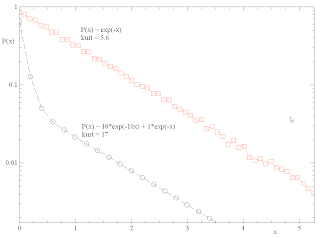The numerical kurtosis of the superposition is 17.

This could be relevant for the "spider web model of cytoskeletal fluctuations": An elementary remodeling step in a remote fiber causes a smaller shift of the bead, i.e. the corresponding probability distribution of shifts has a steeper decay. The total shift distribution is a superposition of the different fiber contributions.

## Tuesday, September 15, 2009

###  Cell Invasion: Summary of preliminary results

A. Modelling the cell invasion process

We have developed a set of mathematical models for the invasion process of tumor cell populations into a half-space of collagen gel. In particular, we aimed for a quantitative understanding of the characteristic shape of the invasion profiles, their temporal evolution, and their dependence on the initial cell surface density.

On the microscopic scale, collagen is a highly inhomogeneous fiber network. The detailed procedure by which individual tumor cells migrate through this porous network (involving steps such as finding adhesion ligands, forming and disintegrating focal adhesion contacts, up- and down-regulating acto-myosin traction forces, ..) is not well understood at present. Moreover, it depends on experimentally inaccessible local conditions. It is therefore reasonable to describe cell migration as a stochastic process, i.e. essentially as a random walk. The effective diffusion constant of this random walk summarizes the complex interactions of the cell with its surrounding material in a coarse-grained way. For simplicity, we have further assumed that the collagen gel is statistically homogeneous and isotropic, i.e. the diffusion of (isolated, non-cooperating) cells of a certain type is equally fast at any position within the gel and does not depend on the spatial directions.

B. Figures of merit for testing invasion models

The most detailed description of a (real or simulated) invasion experiment consists of a complete 3D trajectory

\vec{R}_n(t)=(x_n(t),y_n(t),z_n(t))

for each individual cell n. So far, we have mainly focused on the z-coordinates.

In analogy to the time-averaged "mean squared displacement", a standard tool for analyzing stationary random walks, we can define a population-averaged "mean squared invasion depth":

\left\langle z^2 \right\rangle (t) = (1/N) \; \sum_{n=1}^N (z_n(t))^2,

where n runs over all N cells of the population.

The time-dependent z-density distribution (unit: 1/m)) is defined by

n(z,t) = \sum_{n=1}^N \delta(z-z_n(t)).

Since n(z,t) is usually a very noisy quantity, we work in the following with the (equivalent) cumulative probability distribution

P(z,t) = \int_z^\infty n(z,t) dz\; /\; \int_0^\infty n(z,t) dz.

This dimensionless quantity can be interpreted as the probability to find a cell at depth z, or deeper. For any fixed time t_0, the function P(z,t_0) starts with the value 1 at the top surface (z=0) of the collagen slice and monotonically decreases towards zero for larger depths z.

C. Experimental key features

Measured cumulative probability distributions reveal a large variability, depending on the cell type and on the material parameters of the used collagen gel. However, there are a few general properties that we observed in almost all cases investigated so far:1.) A typical distribution, for fixed time t_0, consists of up to three distinctive layers, or zones. These zones are most easily visable in a semi-logarithmic plot of P(z,t_0).

2.) Within a narrow layer close to the surface, P(z,t_0) is rapidly decaying, i.e. according to a faster-than-exponential law.

3.) Within a broad intermediate zone, the cummulative probability is decaying exponentially, P(z,t_0) ~ e^(-z/z_0) with a characteristic length scale z_0.

4.) For very large depths, at the "front zone", P(z,t_0) decays apparently according to a faster-than-exponential law. Note that, for all finite populations, there is always a single cell at the foremost front of the distribution. Statistics is becoming extremely poor close to that region.

6.) As time passes, the characteristic length scale z_0 of the exponential zone increases.

7.) The mean squared invasion depth of the cell population is increasing with time t. The functional dependence of on t is non-linear, indicating an anomaleous diffusion process.

8.) The invasion profiles P(z,t) depend strongly on the 2D density of tumor cells initially plated onto the gel surface:

9.) For very small population densities, the surface layer, marked by the rapid drop of cumulative invasion propability, disappears.

10.) For increasingly higher densities, the drop of cumulative probability at small depths becomes more pronounced, indicating a dense aggregate of tumor cells immediately below the surface. Beyond this aggregate, only a relatively small fraction of cells is invading into the deep bulk of the gel. However, the characteristic length scale z_0 of the invaded fraction tends to increase with initial cell density.

D. Various investigated models

We have considered a variety of different invasion models and explored their ability to account for the above experimental key features.

The most basic possibility would be a homogeneous population of non-cooperative tumor cells, where all individuals migrate with the same effective diffusion constant, independently from each other. However, the simple Gaussian invasion profiles, resulting from standard diffusion into a half-space, are clearly inconsistent with the complex layered structure of the measured P(z,t) distributions, in particular with the zone of exponential decay (experimental feature 3).

Heterogeneous cell populations with a spectrum of vastly different diffusion constants could produce close-to-exponential cumulative probabilities. Yet, this requires fine-tuning of the assumed parameter distribution. In addition, heterogeneity cannot account for the observed cell density dependence.

It is well-known that standard diffusion combined with a process of reverse drift, back to the surface, at constant velocity, produces exponential profiles. One then has to explain the biological cause of this slight trend in the random walk of the tumor cells. Among the possibilities are mesoscopic spatial gradients in the distribution of local properties (topological, rheological, or chemical) within the gel. Again, such external causes cannot account for the observed cell density dependence.

We have also considered a model in which the cells themselves emit a chemo-attractant, which independently diffuses within the gel and degrades at a certain rate. For certain parameter ranges, the resulting invasion profiles are indeed consistent with the observations. However, the values of the diffusion constants required for a good fit to the data turned out to be not realistic.

E. The "Density-Dependend Diffusion" model

At present, our best model for the invasion process is based on the assumption of a situation-dependent diffusion constant of the tumor cells. This idea is motivated by the observation of the cell aggregate (experimental feature 10) that formes in a narrow layer below the gel surface when the initial cell surface density was large. Within this layer, neighboring cells are very close to each other. The aggregate remains rather stable over long times, meaning that the diffusion constant of cells in an aggregated state is small. On the other hand, once individual cells escape from the aggregate into the bulk of the gel, their diffusion constant becomes much higher, indicated by the large characteristic length scale of the exponential zone.

We therefore tested a simple model in which the individual cells switch their diffusion constant between a small and a large value, depending on the presence or absence of at least one neighboring cell within a certain detection range. The full model has been implemented as a multi-dimenional Monte-Carlo simulation. Additionally, in a mean field approximation, the corresponding 1D Fokker-Planck equation has been solved numerically.

The three profiles shown in the figure below correspond to three separate invasion experiments, differing only in the initial cell surface density (The red, green and blue curve correspond to a total number of 33, 498 and 4348 cells in the total field of view). In all three cases, the tumor cell positions within the gel have been measured after the same time delay (relative to planting the cells onto the gel surface). The characteristic density dependent changes of the invasion behavior, especially the features 9 and 10 listed above, are clearly visible.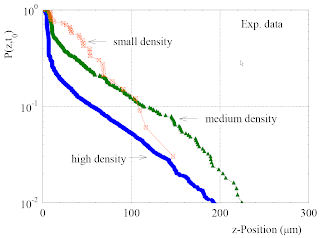The next figure shows the results of corresponding computer experiments, obtained by the Monte Carlo simulation of the DDD model. No attempt has been made to fit the parameters to the specific experiments. Nevertheless, all major features and trends are qualitatively reproduced:## Wednesday, August 12, 2009

###  Cell-Invasion: Implementation of the DDD model

It is possible to solve the Fokker-Planck (FP) equation of post  numerically by direct temporal forward integration, as done before.

Let us discretize space as
z_i = i \; \Delta z

and time as
t_n = n \; \Delta t.

Let \mu be the fraction of density transfered from site i to site i+1 during one time step, so that the corresponding current would be

J_{i,i\!+\!1} = \mu \; \rho_i

and let us denote the density in site i at time n as

\rho^{n}_i = \rho(z_i,t_n).

Except for the most left (i=0) and the most right (i=i_mx) sites (where boundary conditions have to applied properly), the discretized diffusion equation reads

\rho^{n+1}_i  = (1-2\mu) \rho^{n}_i + \mu (\rho^{n}_{i-1} + \rho^{n}_{i+1}).

Note that the physical diffusion constant is related to the discretization parameters as

D = \frac{\mu \Delta z^2}{\Delta t}.

To avoid numerical instabilities, one can set \mu=0.1 and choose a proper time step.

We shall later apply the above method to the DDD model. In this case, \mu is locally replaced by
\mu \rightarrow \mu_i = \mu(\rho^{n}_i).

As a simple test of the method, we start with ordinary diffusion in a box of width 100 with hard walls at z=0 and z=100. The initial density at t=0 is a narrow Gaussian, centered in the middle of the box. The diffusion constant was set to 10.

In a semilog plot, one gets the expected evolution of density: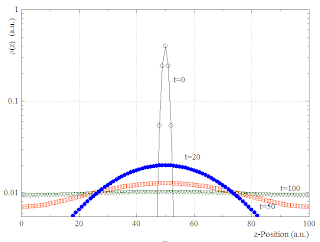The variance of z-distributions follows initially the behaviour of free diffusion. Later it saturates because of the confinement in the box:Next we turn to the model with density dependent diffusion constant (DDD model). The initial density is now localized in the z=0 layer. The evolution of the particle densities, as resulting from numerical integration of the FP equation, looks as follows:In the semilog. plot one can see the characteristic bi-phasic behaviour and the almost exponential regime at intermediate z.

Here the corresponding cummulative probabilities (= invasion profiles):The variance as a function of time ...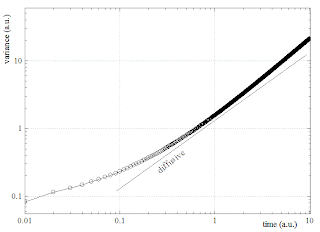... starts sub-diffusively and later appears to become slightly super-diffusive.

###  Cell-Invasion : Clustering : Density Dependent Diffusion (DDD) Model

It would be convenient to formulate the clustering effect in the form of a 1D Fokker-Planck equation that can be quickly solved numerically. For that purpose, remember that the main point of the clustering effect is the simultaneous presence of fast and slow diffusing particles at any depth z. Assume we know the fraction P_NC of particles at z which have no closeby partner within their interaction range r_c. Then we can define a local effective diffusion constant as

D_{eff} =  P_{NC} D_{fast} + (1-P_{NC}) D_{slow}.

Consider a small stripe of material around depth z. Let the local 3D particle density within this z-stripe be rho. We neglect any possible correlations between the positions of different particles within the stripe, i.e. we assume spatial Poisson statistics with average density rho. Then the fraction P_NC is the probability that, for an arbitrary particle, the nearest neighbor is found in a distance larger than r_c. The average number of particles in a sphere of radius r_c is given by

\overline{n} = \rho \;\frac{4}{3}\pi r^3_c.

According to Poisson statistics, the probability that n=0 is

P_{NC} = e^{-\overline{n}} = e^{ - \rho \;\frac{4}{3}\pi r^3_c} = e^{- \rho / \rho_c},

with

\rho_c = \frac{3}{4 \pi}\; r^{-3}_c.

Thus we can define a density dependent diffusion constant:

D_{eff}(\rho) \;= \; (e^{-\rho / \rho_c})  D_{fast} \;+\; (1-e^{-\rho / \rho_c}) D_{slow}.

The figure below shows an example: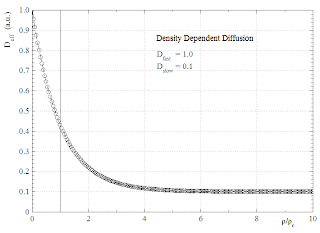Using this, we can write our model as a non-linear Fokker-Planck equation for the position and time-dependent particle density:

\frac{d}{dt} \rho(z,t)\; = \;D_{eff}(\rho(z))\; \frac{\partial^2}{\partial x^2} \;\rho(z,t).

## Monday, August 10, 2009

###  Cell-Invasion : Clustering : Bi-phasic invasion profiles

Another interesting feature of the clustering effect (compare posts  and ) is the emergence of two relatively distinct phases in the invasion profiles:

Close to the cell monolayer at z=0, the cell density is high, so that clusters have a high probability. This leads to a small average diffusion constant and, thus, a weakly invasive phase close to the surface.

At some point, the absolute cell density and the corresponding cluster probability fall below the critical value and most cells switch to a high diffusion constant. A strongly invasive phase is formed further in the bulk.

The following MC simulation demonstrates this effect:Remarkably, many of the measured invasion profiles show qualitatively such a bi-phasic behaviour:Note that for technical reasons the coordinate of the monolayer is not at z=0. No attempt has been made to fit the data.

--------------

Some side remark: As mentioned already in , the value P_cum[z=1] can be interpreted as the invaded fraction of cells. When we zoom into the simulation result above, we can see that this fraction increases from about 0.63 to 0.86 within the time interval between t=20 and t=100 units.###  Cell-Invasion : Slow Layer Effect

The cell clustering effect, as described in posts  and , is not easy to treat analytically. A much simpler, but somewhat similar model is the "slow layer effect":

The particles (tumor cells) undergo standard diffusion (with rates Rb) in the whole bulk of the material. Only in the z=0-layer the diffusion constant (i.e. the transfer rates for intra layer motion and for steps out of the layer) is reduced to a value Rl smaller than Rb.

Before we go into simulations, a simple one-dimensional consideration shows that the abrupt change of rates at z=0 implies a sudden drop of particle density. We have for the temporal change of density \rho_0 at z=0:

\frac{d}{dt}\rho = -\rho R_l + \rho R_b

If we assume a quasi-steady state, where rho and rho change very slowly with time, we obtain

\frac{\rho}{\rho} = \frac{R_l}{R_b},

and thus rho is much smaller than rho, if R_l is much smaller than R_b.

The following figure shows simulation results for the invasion profiles (cummulative probabilities) in a linear plot. The ratio R_l/R_b was 1/1000. The curves correspond to different times (100,200,...,500 units):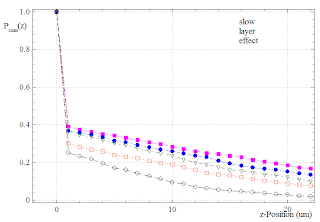One can clearly see the drop of density immediately above the z=0-layer. Note that P_cum acan be interpreted as the fraction of particles that have separated from the boundary layer and invaded the bulk. We might call this quantity the "invaded fraction". It is about 0.4 in the magenta-colored profile above.

A semilog plot of the same results shows that effects of the slow layer range far into the bulk: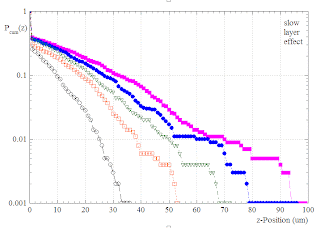The shape of P_cum(z) is slightly different from the case of standard diffusion in . The initial "slope" of the curves appears steeper.

Actually, if one removes the single data point at z=0, P_cum(z) can very well be fitted (in the whole bulk z=1...zMax) by shifted Gaussians, using 3 free parameters P_0, z_0 and \sigma:

P_{cum}(z>1) = P_0 \; e^{-(z+z_0)^2/\sigma^2}Basically this means that the curves are approximately factors of exponentials and Gaussians. For not too large z, the exponential factor will dominate. The exponential range should grow with sigma. Therefore, the larger the bulk diffusion constant, the invasion profiles should appear more and more exponential-like. Hence, even the simple "slow layer" model can to some extent account for exponential invasion profiles.

Is it possible to treat this model analytically in 1D ? I guess that in the limit of smaller and smaller width of the z-slices, the effect of the slow layer translates into a simple boundary value condition for the derivative of rho(z). Except this unusual boundary condition, ones has only to solve a standard diffusion Fokker-Planck equation in the bulk.

I tried a very simple numeric solution of the FP equation, using the methods of an old post (Program: Invade1):Note that this is the real particle density P(z), not the cummulative invasion profile. The density jump corresponds to the expected value Rl/Rb. One can also see that the initial slope of the bulk density curves is negative.

Finally, the corresponding cumulative invasion profile: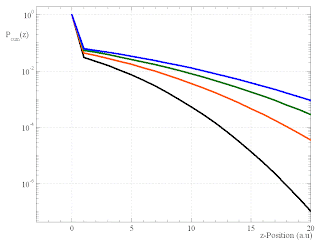## Sunday, August 9, 2009

###  Cell-Invasion : Clustering causes fractional diffusion behaviour ?

One of the most used quantities for the characterization of random walks is the Mean Squared Displacement (MSD) as a function of lagtime, averaged over absolute time. In the case of cell invasion (which might be non-stationary and therefore not suitable for being analyzed with standard MSD), we can define similar quantities of interest as population averages of (powers of) the z-coordinate versus absolute time. For example, we might consider

the Mean Invasion Depth (MID):

\overline{z}(t)=\left\langle z \right\rangle_{pop}=\frac{1}{N}\sum_{n=1}^N z_n(t),

where index n runs over all N agents,

the Mean Squared Invasion Depth (MSID):

\overline{z^2}(t)=\left\langle z^2 \right\rangle_{pop}=\frac{1}{N}\sum_{n=1}^N z^2_n(t),

the Variance (var):

\mbox{var}(t)=\left\langle (z-\overline{z})^2 \right\rangle_{pop}=\frac{1}{N}\sum_{n=1}^N (z_n-\overline{z})^2(t),

and the Invasion Front Position (IFP):

z_{front}(t)=\mbox{max}\left\{ z_n(t) \right\}_{n=1\ldots N}.

Some of these quantities are evaluated in the following for the cell invasion model presented in the last post . We first look at the case of independent random walkers: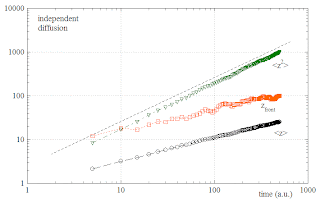In the double-logarithmic plot, the MSID is found to grow linearly with time, as would be expected for normal diffusion. The MID, accordingly, grows with the square root of time. The front particle is always ahead of the mean, but is advancing with the same square-root law.

Now we turn to the invasion with clustering: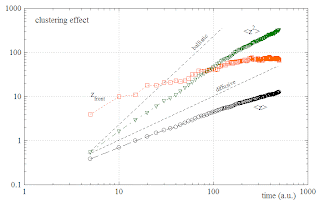Surprisingly, the MSID grows with time as a fractional, super-diffusive power law ! The MID also grows faster than with the square root of time.

Isn't that counter-intuitive ? How can the occasional slowing down of clustered agents lead, effectively, to a "faster-than-diffusive" invasion dynamics ?

A closer look at the figures reveals that, within the first 100 time units, the normally diffusing agents have invaded - on average - further than the clustering agents, in accordance with common sense. Nevertheless, due to the higher power-law exponent of the clustering agents, there would be a cross-over at some later time. However, the MID and the MSID in the model with clustering seem to show a change of behaviour after about 100 time units. They then seem to return to normal diffusive behaviour, thus avoiding a cross-over.

So the super-diffusion is only a transient effect. It remains surprising, at least for me.

## Saturday, August 8, 2009

###  Cell-Invasion : The clustering effect

Last week I had a nice collaboration with Sebastian Probst. He had a very interesting idea:

Assume the agents have a tendency to form and stabilize clusters: Each time they happen to find another closeby agent on their random walk, they drastically reduce their diffusion constant. They won't stop moving entirely, but let's assume they slow down whenever they are in happy company. How would that clustering effect change the invasion process ?

In order to model this idea, we first start again with the simple diffusion simulation from the last post . In regular time intervalls of 100 units, we count how many agents are found within each z-stripe, resulting in a histogram N(z). From this histogram we compute a cumulative probability distribution P_cum(z), which gives the probability that an agent is found in depth z of the material or deeper. In the following, we call this cumulative distributions the invasion profiles.

One finds for the simple diffusion
P_cum(z)-curves which have a negative curvature and approximately Gaussian shapes with a variance that grows with time. Note that this is a semi-log plot, so true Gaussians would show up as downward parabolas.Now we leave everything as before, except that the clustering effect is additionally implemented. For this purpose, we count the number of direct neighbors of each agent. If this number is larger than zero for certain agents, we reduce the hopping rates of these specific agents by a factor of 10 during the next MC cycle. Again we plot the invasion profiles: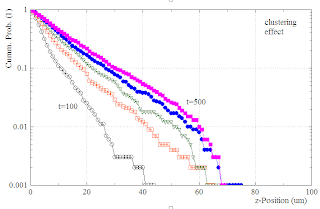The result is remarkable:

As a consequence of the clustering effect, the profiles are changed from about Gaussian to almost exponential shape (showing up as linear lines in the semi-log plot).

This offers a completely new, simple, and testable mechanism for explaining the mostly exponential invasion profiles observed in the experiments. It does not require any explicit assumptions about the diffusion of chemical signalling molecules. The only parameters are the maximum diffusion constant of the agents, their detection range for sensing closeby fellows, and the reduced diffusion constant in the clustering mode.

Future tests:

• The initial density of the cells in the monolayer should drastically affect the invasion process. A small density will reduce the probability of cluster formation and thus drive the system back to the normal, Gaussian diffusion of the agents.

###  Cell-Invasion : 2D Monte-Carlo simulation

We have implemented a Monte-Carlo simulation program for studying tumor cell invasion into a half space of complex bio-material (as a part of a new project described in a recent post and its followups). In the following, the tumor cells are treated as active agents in a multi agent system.

• Space is discretized as a 2D rectangular grid of sites (x,z). The positive z-direction corresponds to the invasion direction, pointing into the material.
• Each site can be occupied by at most one agent. Multiple occupation is forbidden (in a way that is analogous to the Hubbard model used in the theory of metal insulator transitions).
• Each site is assigned a potential energy U(z,x), reflecting the difficulty for an agent to occupy this site. It would be possible, in principle, to investigate potential landscapes of arbitrary complexity. Simple choices would be a completely flat potential, or a binary valued potential without spatial correlations, i.e. binary white noise. The two possible values of the local potential could be zero (no obstacle) and infinity (impenetrable obstacle). And so on.
• There is a certain probability per unit time that an agent moves from its present site to a neighboring site (This leads formally to a kind of "hopping rate", although the agents are thought to move continuously in reality. It is the discrete, "pixelized" detection of their position that implies discrete hopping steps). The maximum hopping rate is R0. However, when the agent moves from a site of low to a site of high potential energy, the hopping rate is reduced by a Boltzmann factor with some effective temperature (Of course, tumor cell invasion is not driven by thermal fluctuations, but by active motor forces. Nevertheless, the cell migration rate will slow down when the cell tries to move into obstacles, i.e. when it enters high energy sites in the potential landscape. This effect can be conveniently modelled by the Boltzmann factor.)
• For the horizontal (z) and vertical (x) edges of the simulation area, boundary conditions can be chosen between hard walls and periodic in our implementation.
• Many agents can be in the system simultaneously. They can interact with each other (in order to describe processes such as binding of tumor cells etc.). They can also change the potential landscape (describing, for instance, stigmergic effects such as the protolysis of collagen by chemicals emitted by the tumor cells).
• The Monte-Carlo algorithm is "exact": In each cycle, a complete list of all possible processes (all possible hops of all agents, etc.) is generated and the rates of these processes (depending on the current system state) are computed, as well as the sum of all rates, which determines the average waiting time dtMean until the next process happens. The MC algoritm then chooses a random, exponentially distributed waiting time according to dtMean. It also chooses one of the processes from the list, with the correct relative probabilities, according to the rates. The process chosen is executed, the system state is updated, the relevant variables are recorded, and the next cycle starts.

As a simple example, let us consider a MC simulation on a grid of 1000(x) times 100(z) sites. In the x-direction, we chose periodic boundaries, but in the z-direction the boundaries are hard (This is realized by placing an almost infinitely high potential wall just outside and along the west and east edge of the potential landscape). Initially, 1000 cells are placed onto the west surface (z=0), forming a complete monolayer. The cells then start to diffuse randomly (This is simply achieved by a flat potential landscape U(z,x)=const. inside the whole simulation area. Then the hopping rates are everywhere homogeneous and isotropic). The picture below shows a snapshot of the distribution of agents over the simulation area after 100 time units (black circles) and after 500 time units (red squares). One can see how the agents are invading the half space.## Tuesday, July 21, 2009

###  Interpretation of the directional correlation function

In post  on random walks with directional persistence, we have defined the correlation function of the direction vectors as
C_{mn}=C_{m-n}=\left\langle \vec{e}_m  \vec{e}_n \right\rangle = \left\langle \cos(\phi_m-\phi_n) \right\rangle,
where the brackets denote the ensemble average. We note that
 C_1 = \left\langle \cos(\phi_{m+1}-\phi_m) \right\rangle. 
The quantity
 \Delta \Phi_1=\phi_{m+1}-\phi_m 
is the so-called turning angle, i.e. the angle between the directions of two successive moves of the random walker. Therefore, the value of correlation function at lagtime 1 is just the average of the cosine of the turning angle. If the probability distribution of the turning angle is denoted by
 P(\Delta \Phi_1), 
we thus have
 C_1 = \int_{-\pi}^{\pi} d\Delta \Phi_1 \;P(\Delta \Phi_1) \; \cos(\Delta \Phi_1).  
In earlier publications, researchers have defined an index of persistence by
 p_{\Phi_1} = 2 \left( \int_{-\pi/2}^{\pi/2} d\Delta \Phi_1 \;P(\Delta \Phi_1)\right) -1. 
This can be rewritten in the form
  p_{\Phi_1} = \int_{-\pi}^{\pi} d\Delta \Phi_1 \;P(\Delta \Phi_1)\; g(\Delta \Phi_1),
where the weight function g() has the constant value +1 in the central interval [-pi/2...+pi/2] and the value -1 in the remaining intervals [-pi...-pi/2] and [+pi/2..+pi]. It is clear that this weight function can be equally well be replaced by the smooth cosine function above. Therefore, we learn that the index of persistence is basically the value of the directional correlation function at lagtime 1:
 p_{\Phi_1} \approx C_1.
In an analogous way, the correlation function at higher lagtimes N>1 is just the index of persistence at the corresponding lagtime N:
 p_{\Phi_N} \approx C_N, 
provided that the turning angles are properly defined as
 \Delta \Phi_N = \phi_{m+N}-\phi_m. 

## Monday, July 20, 2009

###  MSD of random walks with given SWD and directional correlations

We consider a stationary random walk of a particle in 2D. The position of the particle is measured at regular time intervalls
t_n=n\Delta t \; \mbox{with} \; n=0,1,\ldots
After each intervall, the particle has moved by one step, characterized by a positive step width and a direction (unit) vector:
\Delta\vec{R}_n = s_n\;\vec{e}_n = s_n \; \left( \cos(\phi_n) , \sin(\phi_n) \right) .
Let the probability distribution function of the step widths (abbreviated by SWD) be given by
P(s) = \mbox{Prob}(s_n=s)
with finite mean and variance and assume that the successive step widths are statistically independent random variables.
\left\langle (s_m-\overline{s})(s_n-\overline{s})\right\rangle = \overline{s^2}\delta_{mn}.
Let the correlation function of the direction vectors be defined as
C_{mn}=\left\langle \vec{e}_m  \vec{e}_n \right\rangle = \left\langle \cos(\phi_m-\phi_n) \right\rangle,
where the brackets denote the ensemble average. Assume that the width and direction of each step are statistically uncorrelated.
\left\langle (s_m-\overline{s})\vec{e}_n\right\rangle = \vec{0}.
The position of the particle at time step N is given by
\vec{R}_N = \sum_n \Delta\vec{R}_n.

What is the ensemble averaged mean squared displacement (MSD) of the particle ?

We have
\left\langle \vec{R}^2_N  \right\rangle = \left\langle  \sum_m \sum_n \Delta\vec{R}_m \Delta\vec{R}_n \right\rangle 

=\sum_{m,n} \left\langle s_m\vec{e}_m\;s_n\vec{e}_n \right\rangle 

Due to the independence of step lengths and directions this factorizes to
=\sum_{m,n}  \left\langle s_m s_n \right\rangle \; \left\langle \vec{e}_m \vec{e}_n  \right\rangle

=\sum_{m,n} \left\langle s_m s_n \right\rangle \; C_{mn}.

We next write the step widths in the form of average plus fluctuation:
s_m = \overline{s} + \Delta s_m,
understanding that the fluctuations are zero mean:
\left\langle \Delta s_m \right\rangle = 0.
This yields
\left\langle \vec{R}^2_N  \right\rangle = \sum_{m,n} C_{mn} \left\langle (\overline{s} + \Delta s_m) (\overline{s} + \Delta s_n) \right\rangle.
Under our statistical assumptions, we have
\left\langle (\overline{s} + \Delta s_m) (\overline{s} + \Delta s_n) \right\rangle = \overline{s}^2 + \left\langle \Delta s_m \Delta s_n \right\rangle =   \overline{s}^2 + \overline{s^2}\;\delta_{mn},
Note that here
 \overline{s^2} 
stands for the variance of the fluctuation of the step widths. We obtain
\left\langle \vec{R}^2_N  \right\rangle = \overline{s}^2\sum_{mn}C_{mn}+\overline{s^2}\sum_m C_{mm}.

Since
 C_{mm}=\left\langle \vec{e}^2_m \right\rangle = 1,

we obtain
 \left\langle \vec{R}^2_N  \right\rangle = \overline{s}^2\sum_{mn}C_{mn}+N\;\overline{s^2}.
Because our random walk was assumed to be a stationary random process, the correlation functions can only depend on time differences,
 C_{mn}=C_{m-n}
, and thus we have
 \left\langle \vec{R}^2_N  \right\rangle = N\;\overline{s^2} + \overline{s}^2\sum_{mn}C_{m-n}.

It is easy to show that
 \sum_{m=1}^N \sum_{n=1}^N f_{(m-n)}=\sum_{k=-N+1}^{N-1}(N-k)  f_k.

Using this, we arrive at
 \left\langle \vec{R}^2_N  \right\rangle = \overline{s^2}\;N + \overline{s}^2\sum_{k=-N+1}^{N-1}(N-k)C_k
 =\overline{s^2}\;N + N\overline{s}^2\sum_{k=-N+1}^{N-1}C_k - \overline{s}^2\sum_{k=-N+1}^{N-1}k C_k.
Since the correlation function is symmetric with respect to k, while k is asymmetric, the last term vanishes, yielding
 \left\langle \vec{R}^2_N  \right\rangle = \overline{s^2}\;N + \overline{s}^2\; N\;\sum_{k=-N+1}^{N-1}C_k.

In the sum, we can further extract the k=0 term
C_0=1
and make use of the symmetry
C_{-k}=C_k
to obtain
\left\langle \vec{R}^2_N  \right\rangle = \overline{s^2}\;N + \overline{s}^2\;N + \overline{s}^2\; 2N\;\sum_{k=1}^{N-1}C_k.
Thus we finally arrive at
\left\langle \vec{R}^2_N  \right\rangle = \left( \overline{s^2}+ \overline{s}^2+ 2\overline{s}^2\sum_{k=1}^{N-1}C_k\right) N.

We see that the MSD of our particle is completely determined by the mean and variance of the step width distribution and by the directional correlation function. All step width distributions with the same mean(s) and var(s) produce the same MSD. Moreover, interesting (non-diffusive) MSD-versus-lagtime relations can only be generated via the directional correlations. We have excluded the possibility of Levi flights and the like by demanding that the SWD is not heavy-tailed.

Note to self: There are at least 4 possibilities how fractional powerlaw MSDs can arise: (1) heavy-tailed step width, (2) heavy-tailed waiting times, (3) persistent directions, or (4) long-time correlated statistical dependencies between the widths and directions of the steps. It might be interesting to follow possibility (4), if I should ever find the time.

We can check a few limiting cases:

(a) Brownian diffusion

In this case
 C_k=\delta_{k0}, 
so that
 \sum_{k=1}^{N-1}C_k = 0 
and, hence,
 \left\langle \vec{R}^2_N  \right\rangle = (\overline{s}^2 + \overline{s^2})\;N,
as it should be for a diffusive process. Note that for an exponential step width distribution,
\overline{s}^2 = \overline{s^2},
so that
 \left\langle \vec{R}^2_N  \right\rangle = 2 \overline{s}^2 N.

(b) Ballistic motion

In this case
 \phi_m=\phi_n=\phi, 
so that we get
 \cos(\phi_m-\phi_n)=\cos(0)=1 
and so
 C_{mn}=1=C_k 
and so
 \sum_{1}^{N-1}C_k = (N-1) 
and so
\left\langle \vec{R}^2_N  \right\rangle = \left( \overline{s^2}+ \overline{s}^2+ 2\overline{s}^2 (N-1)\right) N.
In the limit of large N we obtain
\left\langle \vec{R}^2_N  \right\rangle = 2\overline{s}^2 N^2,
i.e. the MSD grows quadratically with time, as expected for ballistic motion.

(c) Exponentially decaying directional correlations

In this case,
C_k=q^{-k}.
For a measuring time intervall dt and a physical decorrelation time \tau, we would have to set
 q=e^{\Delta t/\tau}.
The sum can be written as
\sum_{k=1}^{N-1}C_k = -1 + \sum_{k=0}^{N-1} (q^{-1})^k = -1 +\frac{1-q^{-N}}{1-q}, 
so that
\left\langle \vec{R}^2_N  \right\rangle = \left( \overline{s^2}+ \overline{s}^2+ 2\overline{s}^2 (-1 +\frac{1-q^{-N}}{1-q}) \right) N.
=\left( \overline{s^2}- \overline{s}^2+ 2\overline{s}^2 \frac{1-q^{-N}}{1-q} \right) N. 
Note that for
N\Delta t/\tau<<1
we have
 1-q^{-N}=1-e^{-N\Delta t/\tau}\approx 1-1+N\Delta t/\tau=N\Delta t/\tau=N \ln(q).
So for lagtimes smaller than the decorrelation time, the MSD is ballistic, for larger lagtimes diffusive, as it should be.

(c) Long-time correlated directional correlations

We next assume that for large lagtimes, the correlations decac according to a power law
C_k\rightarrow c_0 N^{-\gamma} = c_0 (t/\Delta t)^{-\gamma}.

To treat this case, we change from discrete time steps to a continuous time by replacing
 N\rightarrow t/\Delta t
and
\sum_{k=1}^{N-1}C_k \rightarrow \frac{1}{\Delta t}\int_{t0}^t C(t^{\prime})dt^{\prime},
to obtain
\left\langle \vec{R}^2(t)  \right\rangle = \left( \overline{s^2}+ \overline{s}^2+ \frac{2\overline{s}^2}{\Delta t}\int_{t0}^t C(t^{\prime})dt^{\prime}\right)t/\Delta t .
In the limit of large lagtimes we have
\int_{t0}^t C(t^{\prime})dt^{\prime} = \int_{t0}^t c_0(t^{\prime}/\Delta t)^{-\gamma}dt^{\prime} \rightarrow (t/\Delta t)^{1-\gamma},
so that
\left\langle \vec{R}^2(t)  \right\rangle \rightarrow R_0^2\;(t/\Delta t)^{2-\gamma} .
For example, directional correlations decaying with lagtime as an inverse square root will produce a MSD growing with a fractional powerlaw exponent of 1.5.

## Wednesday, July 15, 2009

### Stochastic Process Generator with arbitrary PDF and ACF

Such an algorithm would be very useful for many purposes. Some ideas on this problem have been published by Primak.

Here some inefficient, but general concept:

First, a large number of independent sample values x_n (n=1...N) are generated, in accordance with the prescribed probability distribution function ( PDF, P(x) ). If the x_n would be stringed together in their original, independently generated sequence, the autocorrelation function ( ACF, Cxx(t) ) would be that of white noise. However, if the N sample values are re-shuffled in the right way (i.e. if a permutation is applied to the order of values), the ACF will change, while the PDF remains constant. It is therefore possible to use an evolutionary optimization algorithm for the re-shuffling procedure, which tries to mutate the order of the sample values until the ACF matches best the goal Cxx(t). The elementary mutations could, for example, consist of the exchange of compact intervalls of sample values.

A further Google-search yields the following, related papers:
• Generation of pseudo random processes with given marginal distribution and autocorrelation function ( A. S. Rodionov et al. (2009))
• Windowing to simultaneously achieve arbitrary autocorrelation characteristics and probability densities in noise generators ( R. Taori et al. )

### New paper accepted for publication in PRE

Title: "Noise and critical phenomena in biochemical signaling cycles at small molecule numbers"

Authors: C. Metzner , M. Sajitz-Hermstein , M. Schmidberger , B. Fabry

Abstract: Biochemical reaction networks in living cells usually involve reversible covalent modification of signaling molecules, such as protein phosphorylation. Under conditions of small molecule numbers, as is frequently the case in living cells, mass action theory fails to describe the dynamics of such systems. Instead, the biochemical reactions must be treated as stochastic processes that intrinsically generate concentration fluctuations of the chemicals. We investigate the stochastic reaction kinetics of covalent modification cycles (CMCs) by analytical modeling and numerically exact Monte-Carlo simulation of the temporally fluctuating concentration. Depending on the parameter regime, we find for the probability density of the concentration qualitatively distinct classes of distribution functions, including power law distributions with a fractional and tunable exponent. These findings challenge the traditional view of biochemical control networks as deterministic computational systems and suggest that CMCs in cells can function as versatile and tunable noise generators.

Here is the PDF of the final version on arxiv.

## Saturday, July 4, 2009

### John Holland: Hidden Order

I started to read John H. Holland's book "Hidden Order - How Adaption Builds Complexity". A few major ideas of this work will be summarized in the following post.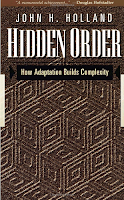Cities, immune systems, central nervous systems and ecosystems as examples of Complex Adaptive Systems (CAS). CAS consist of interacting agents. Each agent is described by stimulus-response rules. Clusters of rules can generate any behaviour that can be computationally described. The range of available stimuli and responses determines the set of possible rules. Agents adapt to their environment by changing their stimulus-response rules based on experience: Learning. A large part of the environment consists of other agents.

There are 7 basic properties or mechanisms common to all CAS:

• Aggregation
In the first sense: In order to model a system, we aggregate similar things into categories and then treat them as equivalent. In the second sense: Agents aggregate to meta-agents with new emergent properties, giving rise to hierarchical structures typical of CAS.

• Tagging
Often several agents in a system appear indistinguishable from the outside, i.e. without closer interaction their differences are hidden. The CAS can break this symmetry of the semi-identical objects by tagging them (e.g., writing symbols on identical looking white balls). Also, different looking objects can be tagged as identical for other purposes. Tagging facilitates selective interactions and thus provides a basis for filtering, specialization, cooperation and hierarchical structure formation.

• Nonlinearity
Using standard definition of linear function f(x,y,z..) = ax + by + cz +... Example for nonlinear interaction: Bimolecular reaction rates, such as f(x,y) = d/dt z(t) = a x(t)y(t). Nonlinearities typically prevent mathematical aggregation (model simplification by coarse-graining). This section of Holland's book is slightly confusing, in my opinion. In order to demonstrate the impossibility of coarse-graining a nonlinear system in the same way as a corresponding linear system, I would prefer an example such as this.

• Flows
Typically, a CAS has a network structure of nodes (the agents) and links (the selective connections between agents). The dynamics of the CAS consists, beside the occasional addition or removal of nodes and links, mainly in a flow of some "material" (bio mass, chemicals, signals, goods, money, ..) through this network. The agents process the incoming flux of material into new forms and distribute it to their output nodes. Holland mentiones two general effects connected to flows. First, the "multiplier effect": If one injects additional material into a specific node, this node will typically retain a part of the extra amount and pass on a fraction r to its output nodes. In a linear chain of identical agents, the total change in the system created by the injection would be 1+r+r^2+r^3+... = 1/(1-r) > 1. So the initial change is multiplied. Second, the "recycling effect": If extra material is injected into a node that is part of a (sub-) network with loops, then the circling of the material can further increase the total effect dramatically. Also, due to circling the material is remaining longer within the system (Example: Food webs in rain forests).

• Diversity
Diversity, i.e. the emergence of ever different types of agents and interactions, comes about because the agents actively try to find and occupy new niches by adaption (mutation and selection). After removing an agent, such niches are automatically refilled by similar agents (persistent patterns). With each new type of agent, further opportunities for interactions are created. In systems with recycling the number of opportunities for participation are particularly large.

• Internal Models
Complex agents learn to behave in a given environment in a way that increases the probability of future reward. This requires to build an internal model of (relevant aspects of) the world, allowing to predict the results of actions. Models can be tacit (hardwired into the agent's stimulus-response machinery) or overt (explicit internal comparison of alternatives). They can be improved by adaption (variation and selection).

• Building Blocks
CAS in complex environments have to respond to ever changing situations. They deal with this variety by decomposing novel situations into simpler, re-occuring and thus reusable building blocks. With a modest repertoire of nA variants of type A building blocks and nB variants of type B building blocks one can already compose nA*nB different composed objects, so this method is extremely efficient.

## Wednesday, May 27, 2009

### Emergence of long-time correlations in reaction networks - A concept

I am posting here some preliminary ideas on the design of a biochemical reaction network that produces concentration pulse trains with a powerlaw distribution of pulse widths. I don't aim to find the most elegant solution, a proof of principle would be ok.

The idea is to utilize the intrinsic randomness of single-molecule reactions and to amplify it into macroscopic population fluctuations using nonlinear effects. The concept goes as follows:

There is a huge reservoir of some substance X0. It can be converted into another molecular species X by the help of an (activated) growth factor G*. Another enzyme, called the decay factor D, reconverts X back into X0. The growth reaction is assumed to be autocatalytic:

X0 + X + G* --> 2X + G*

For the decay reaction we assume that the enzyme D is operating within the saturation regime, i.e. at constant conversion rate:

X + D --> X0 + D.

At the beginning of each pulse, the autocatalytic growth reaction produces an exponential "concentration explosion", as long as the activated growth factor G* is present. If we assume, in the extreme case, that we have only a single G*-molecule around which looses its activation status spontaneously (Poisson process)

G* --> G,

we have the exponential growth of X terminated after an exponentially distributed time interval DeltaT_grow. This creates a power-law-distributed maximum concentration of X. After this event, the decay reaction reduces the concentration X at constant rate. The time period DeltaT_decay until the concentration is back to "ground level" is, therefore, also power-law-distributed. This is the main mechanism to create long-time correlations.

(The decay reaction is already proceeding during the exponential growth phase. But this should be no problem as soon as the growth rate greatly exceeds the decay rate)

Since we want to create a more or less binary concentration pulse, we couple the heavily fluctuating X-concentration to another chemical species E:

X + E <---> XE

Assume that the total number #E_tot of E-molecules is small. Then, as long as the number of available X-molecules is larger than a certain threshold, basically all E will be bound in XE-complexes, i.e. the number of XEs saturates at the limit #E_tot. Vice versa, if the X are below threshold, the number of XEs falls to zero. So the XE-signal has the desired binary pulse character. The "on-time" of XE(t) should be power-law-distributed.

There remains (at least) one problem with this scheme: How is the next pulse initiated ? To do this, we only need to re-activate the growth factor. However, it is important that this does not happen before the last pulse is over, i.e. before X is decayed back to ground level. We therefore couple the activation to the binary switch molecule E/XE:

G + E --> G* + E

E is not available during the period when X is large, because all E is bound into XE-complexes.

## Monday, May 25, 2009

### Cell invasion (6) : First test of chemotactic model

I have implemented the coupled drift-diffusion equations using the simple numerical method tested recently.

For the first tests (program: invade3), the general parameter settings were as follows:

len=100.0;
dz=1.0;
timeSim=1000.0;
TMX=5;

As the initial conditions, the density profile of the guiding substance is set zero g(z,t=0) = 0. The cell density profile c(z,t=0) is set to a half-Gaussian of variance=1, with the maximum at the left boundary.

Run A: Nonmoving, insensitive cells. Effect of guide production, diffusion and decay

The cells remain in their initial distribution (because of D_c=0), sharply localized at the left boundary.The guiding substance is constantly produced by the stationary cells (almost a point source). It also diffuses and decays. This leads quickly to a stationary, exponential concentration profile:Run B: Additional slow cell diffusion, no sensitivity

Keeping all other parameters constant, the cell diffusion constant is next set to D_c=0.1. The cells, being not sensitive to the guide, now show the expected Gaussian diffusion profiles:The initially exponential profile of the guiding substance is now gradually transforming into a Gaussian profile. This is due to the finite memory of the guide distribution and its constant re-production by the cells, which themselves assume a Gaussian distribution:Correction: In the above figure, the green and blue curve corresponds to t=200 and t=400, respectively.

Run C: Adding sensitivity to the cells

Keeping all other parameters as in run B, we now set the sensitivity parameter to sens=3. Interestingly, the cells, starting from the initial Gaussian, now develop an approximately exponential profile:The distribution of the guiding substance develops a non-Gaussian tail as well:Correction: In the above figure, the lowest two curves correspond to t=200 and t=400, respectively.

The preliminary interpretation is as follows: Before the slow cell diffusion is setting in, the faster dynamics of the guiding substance is developing a concentration gradient. This gradient causes an effective back drift of the cells.

Conclusion: The chemotaxis model can produce approximately exponential invasion profiles, as observed in the experiments.

Idea for analytical case:

In the limit of D_g being much larger than D_c, the stationary g(z) should become close to a perfect exponential with a large spatial decay constant. If this decay constant exceeds the width of the stationary cell profile, it can (for those small z) be Taylor-approximated by a linear profile. The gradient of a linear profile is constant, corresponding to backward drift with constant velocity. This can be shown analytically to result in an exponential cell density profile.

It also seems mathematically reasonable that an exponential ansatz for both g(z) and c(z) can self-consistently solve the stationary coupled drift-diffusion equations (containing only derivatives and products of g(z) and c(z)) in the limit D_g>>D_c.

Note that this limit is also biologically reasonable, because small signalling molecules can diffuse much more easily through a dense matrix than the huge living cells.

### Cell invasion (5) : Cooperation via Chemotaxis

Back

It is known that cells can communicate with each other via signalling molecules that are diffusing in the extracellular matrix (e.g. cytokines). Would such chemotaxis be compatible with the apparent backward drift component observed in the motion of invading cells ?

To include chemotaxis into the cell invasion model, we assume that the effective drift velocity is proportional to the local gradient of the "cytokine" concentration. This leads to the following set of coupled equations:

Drift-diffusion of cells:
\frac{\partial}{\partial t}c(z,t) = - \frac{\partial}{\partial z}\left[v(z,t)\;c(z,t)\right] + D_c \frac{\partial^2}{\partial z^2}c(z,t),
v(z,t) = s\; \frac{\partial}{\partial z} g(z,t)
Production, diffusion and decay of the guiding substance:
\frac{\partial}{\partial t}g(z,t) = p\!\cdot\!c(z,t) + D_g \frac{\partial^2}{\partial z^2}g(z,t) - d\!\cdot\!g(z,t)
Here, the two coupled fields are the cell concentration profile c(z,t) and the concentration profile of the guiding substance (the "cytokine") g(z,t). The diffusion constants for cells and guiding substance molecules are denoted by D_c and D_g, respectively. In addition, we introduce a sensitivity parameter s, a production parameter p and a decay parameter d. It is assumed that the guiding substance is locally produced by each cell at a constant rate and that it decays within a characteristic time 1/d.

## Friday, May 22, 2009

### Cell invasion (4) : Numerical solution of drift-diffusion equation

Back

The cell invasion problem requires an accurate numerical solution of coupled partial differential equations. The fields (concentration profiles) f(z,t) will depend on one spatial coordinate z and the time t. The spatial coordinates will be restricted to the intervall [0,len], with well-defined boundary conditions at the left and right limits: The current must dissapear outside the intervall.

I will try to solve this problem by discretizing the z-coordinate into a regular grid,
z_k = k\;\Delta z,

and then treating each z_k as a separate dynamical variable. The problem can thereby be mapped onto a set of coupled ordinary differential equations, which are solved with the Runga-Kutta method.

As a simple test and practice case, consider the drift-diffusion equation
\frac{\partial}{\partial t}c(z,t) = - v \frac{\partial}{\partial z}c(z,t) + D \frac{\partial^2}{\partial z^2}c(z,t),

with position- and time-independent diffusion constant D and drift velocity v.

For the following tests, we set the initial distribution c(z,t) equal to a narrow, normalized Gaussian (variance=10), placed at the center of the intervall. The spatial resolution is set to dz=1.

Test 1: Free diffusion in a box

First we set the drift-velocity equal zero. The initial peak should broaden with time. Asymptotically, a flat distribution should develop, while the integral (total particle number) is preserved. The simulation shows the expected behaviour: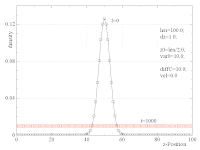Test 2: Diffusion with drift (boundaries far away)

The case of combined diffusion and drift has been compared directly with the analytical solution (solid lines). One expects the center z_c(t) of the Gaussian to move according to
z_c(t) = v\;t

and the variance to grow linearly
\sigma^2(t)=\sigma^2(0)+ 2Dt

The simulation (dashed lines) fits the analytical curves nicely, even for the modest spatial resolution of dz=1:Test 3: Diffusion with drift against a wall

Finally, we choose a negative drift velocity and wait until the distribution is pushed againts the left wall. We now expect the stationary solution
c(z)=\frac{v}{D}\;e^{-(v/D)z}.`

Again, the simulation (symbols) agrees very well with the analytical solution (solid lines):As a conclusion, the simple numerical method seems to work as expected. I have also checked that the results do not change significantly when the resolution dz is reduced.

Next